Next: Three-body problem Up: Rigid body rotation Previous: Cassini's laws

# Exercises

1. Let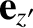,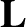, and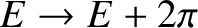be the symmetry axis, the angular momentum vector, and the angular velocity vector, respectively, of a rotating body with an axis of symmetry. Demonstrate that these three vectors are coplanar.

2. Verify Equations (8.51) and (8.52).

3. A rigid body having an axis of symmetry rotates freely about a fixed point under no torques. Ifis the angle between the symmetry axis and the instantaneous axis of rotation, show that the angle between the axis of rotation and the invariable line (thevector) is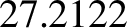where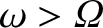(the moment of inertia about the symmetry axis) is greater than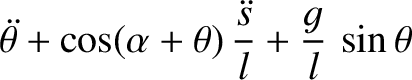(the moment of inertia about an axis normal to the symmetry axis). (From Fowles and Cassiday 2005.)

4. Because the greatest value ofis 2 (symmetrical lamina) show from the previous result that the angle between the angular velocity and angular momentum vectors cannot exceed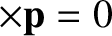. Find the corresponding value of. (Modified from Fowles and Cassiday 2005.)

5. A thin uniform rod of length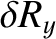and massis constrained to rotate with constant angular velocityabout an axis passing through the center of the rod, and making an anglewith the rod. Show that the angular momentum about the center of the rod is perpendicular to the rod, and is of magnitude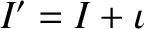. Show that the torque is perpendicular to both the rod and the angular momentum vector, and is of magnitude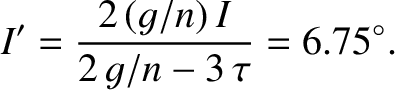. (From Fowles and Cassiday 2005.)

6. A thin uniform disk of radiusand massis constrained to rotate with constant angular velocityabout an axis passing through its center, and making an anglewith the normal to the disk. Find the angular momentum about the center of the disk, as well as the torque acting on the disk.

7. A freely rotating rigid body has principal moments of inertia such that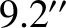.
1. Demonstrate that the rotational energy of the body attains its maximum and minimum values (at fixed) when the body rotates about the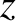- and the-axes, respectively.
2. Demonstrate, from Euler's equations, that the rotational state is stable to small perturbations when the body rotates about either the-axis or the-axis, but unstable when it rotates about the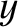-axis.

8. The length of the mean sidereal year, which is defined as the average time required for the Sun to (appear to) complete a full orbit around the Earth, relative to the fixed stars, is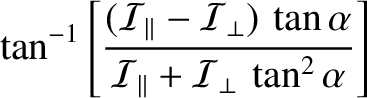days (Yoder 1995). The mean tropical year is defined as the average time interval between successive vernal equinoxes. Demonstrate that, as a consequence of the precession of the equinoxes, whose period is 25,772 years (Yoder 1995), the length of the mean tropical year is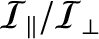minutes shorter than that of the mean sidereal year (i.e.,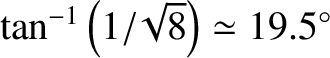days).

9. Consider an artificial satellite in a circular orbit of radiusabout the Earth. Suppose that the normal to the plane of the orbit subtends an angle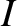with the Earth's axis of rotation. By approximating the orbiting satellite as a uniform ring, demonstrate that the Earth's oblateness causes the plane of the satellite's orbit to precess about the Earth's rotational axis at the approximate rate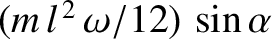Here,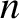is the satellite's orbital angular velocity,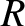is the Earth's mean radius,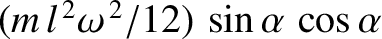,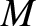is the Earth's mass, andandare the Earth's parallel and perpendicular (to the rotation axis) moments of inertia. Is the precession in the same sense as the orbital motion, or the opposite sense?

10. A Sun-synchronous satellite is one that always passes over a given point on the Earth at the same local solar time. This is achieved by fixing the precession rate of the satellite's orbital plane such that it matches the rate at which the Sun appears to move against the background of the stars. What orbital altitude above the surface of the Earth would such a satellite need to have in order to fly over all latitudes between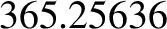N andS? Is the direction of the satellite orbit in the same sense as the Earth's rotation (prograde), or the opposite sense (retrograde)? Note that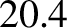for the Earth (Yoder 1995).

11. Consider an aspherical moon in a low eccentricity Keplerian orbit about a spherical planet. Suppose that the moon is locked in a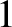:spin-orbit resonance. Demonstrate that, to lowest order in the eccentricity, the optical libration of the moon can be accounted for by saying that the moon's long axis (in the orbital plane) always points toward the empty focus of the orbit.Next: Three-body problem Up: Rigid body rotation Previous: Cassini's laws
Richard Fitzpatrick 2016-03-31